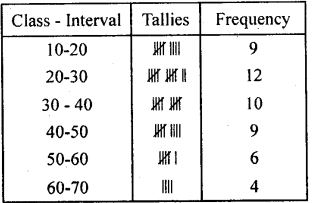# KSEEB Solutions for Class 8 Maths Chapter 13 Statistics Ex 13.1

Students can Download Maths Chapter 13 Statistics Ex 13.1 Questions and Answers, Notes Pdf, KSEEB Solutions for Class 8 Maths helps you to revise the complete Karnataka State Board Syllabus and score more marks in your examinations.

## Karnataka Board Class 8 Maths Chapter 13 Statistics Ex 13.1

Question 1.
The marks scored by 40 candidates in an examination (out of 100) is given below.
75, 65, 57, 50, 32, 54, 75, 67, 75, 88, 80, 42, 40, 41, 34, 78, 43, 61, 42, 46, 68, 52, 43, 49, 59, 49, 67, 34, 33, 87, 97, 47, 46, 54, 48, 45, 51, 47, 41, 43.
Prepare a frequency distribution table with the class size 10. Take the class intervals as 30 – 39, 40 – 49 and answer the following questions.
(i) Which class intervals have highest and lowest frequency.
(ii) Write the upper and lower limits of the class interval 30 – 39.
(iii) What is the range of the given distribution?(i) The class interval 40-49 has highest frequency and 90-99 has the lowest frequency.
(ii) The lower limit of the class interval 30 – 39 is 29.5 and upper limit is 39.5
(iii) Range = Highest score – lowest score = 97- 32 = 65
Range = 65.

Question 2.
Prepare the frequency distribution table for the given set of scores. 39, 16, 30, 37, 53, 15, 16, 60, 58, 26, 28, 19, 20, 12, 14, 24, 59, 21, 57, 38, 25, 36,34, 15, 25, 41, 52, 45, 60, 63, 18, 26, 43, 18, 27, 59, 63, 46, 48, 25, 33, 46, 27, 46, 42, 48, 35, 64, 24 Take class intervals as (10 – 20), (20, 30)…. and answer the following.
(i) What does the frequency corresponding to the third class interval mean?
(ii) What is the size of each class interval? Find the midpoint of the class interval 30 – 40.
(iii) What is the range of the given set of scores?$\frac{(30+40)}{2}$ =$\frac { 70 }{ 2 }$ = 35